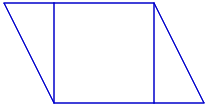### Home > CC2MN > Chapter 10 > Lesson 10.2.3 > Problem10-58

10-58.

Draw a parallelogram with a base of $3$ cm and a height of $7$ cm and show how to calculate its area.

An example of a parallelogram is given below.
It is divided into triangles and a square.
You can use this strategy to find the area of a parallelogram.